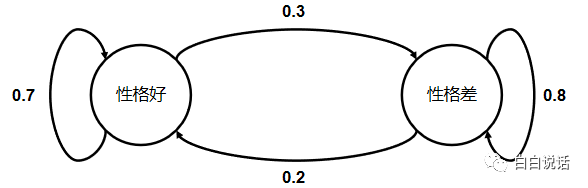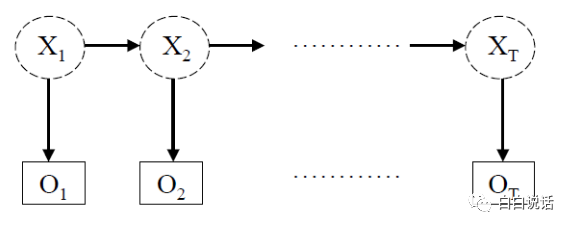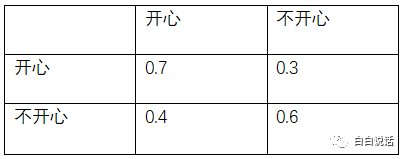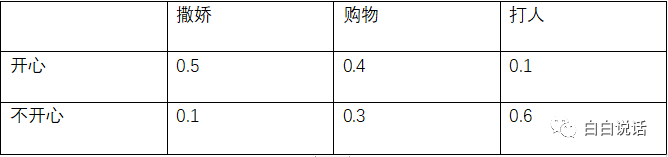## 白白说算法：相亲中的马尔科夫模型

3 评论 3763 浏览 8 收藏 11 分钟## 一、马尔科夫模型1. 状态：性格好、性格差
2. 初始向量：在系统定义时间为0时，状态出现的概率。比如从女儿妈妈出现的时间算起认为是系统的0时，那么她妈妈性格好或性格差的的概率就是初始向量。
3. 状态转移矩阵：上图列出的表格就是状态转移概率，用于描述状态之间的转换。

## 二、隐马尔可夫链### 1. 隐马尔可夫模型主要解决的问题

1. 给定一个模型，求某个观察序列O的概率
2. 给定模型和观察序列O，求可能性最大的X序列
3. 对于给定的观察序列O，调整隐马尔可夫模型的参数，使得观测序列O出现的概率最大• P(第一时刻开心)=P(撒娇|开心)*P(开心|初始情况)=0.5*0.6=0.30
• P(第一时刻不开心)=P(撒娇|不开心)*P(不开心|初始情况)=0.1*0.4=0.04

• P(第一时刻不开心，第二时刻不开心)=P(不开心|第一时刻)*P(不开心->不开心)*P(购物|不开心)=0.04*0.6*0.3=0.0072
• P(第一时刻不开心，第二时刻开心)=P(不开心|第一时刻)*P(不开心->开心)*P(购物|开心)=0.04*0.4*0.4=0.0064
• P(第一时刻开心，第二时刻开心)=P(开心|第一时刻)*P(开心->开心)*P(购物|开心)=0.30*0.7*0.4=0.084
• P(第一时刻开心，第二时刻不开心)=P(开心|第一时刻)*P(开心->不开心)*P(购物|不开心)=0.30*0.3*0.3=0.027

• P(第二时刻不开心,第三时刻不开心)=P(不开心|第二时刻)*P(不开心->不开心)*P(打人|不开心) =0.027*0.6*0.6=0.00972
• P(第二时刻不开心,第三时刻开心)=P(不开心|第二时刻)*P(不开心->开心)*P(打人|开心) =0.027*0.4*0.1=0.00108
• P(第二时刻开心,第三时刻开心)=P(开心|第二时刻)*P(开心->开心)*P(打人|开心) =0.084*0.7*0.1=0.00588
• P(第二时刻开心,第三时刻不开心)=P(开心|第二时刻)*P(开心->不开心)*P(打人|不开心) =0.084*0.3*0.6=0.01512

### #专栏作家#

1. 你妈妈跟女儿的例子，表格弄得一脸懵逼，首列应该是妈妈性格好和妈妈性格差，首行应该是女儿性格好的概率和女儿性格差的概率吧

回复
2. 在我回家计算完之后，发现妹子把我好友删了。

回复
1. 样本数量不够，建议多备点 😐

回复